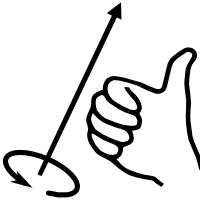# Polyhedron

A polyhedron is the most general 3d Primitive available for openEMS. It can be used to create a body with (nearly) any shape by defining a closed surface using verteces and faces.

## DescriptionThe right hand rule: When using the right hand the thumb indicates the normal direction of the face and the fingers show the direction how to order the vertices for each face.

```CSX = AddPolyhedron(CSX, propName, prio, vertices, faces, varargin)
```

with the following parameters:

• CSX: The original CSX structure
• propName: name of the assigned property
• prio: priority of the primitive
• vertices: cell array of all vertices
• faces: cell array of all faces
• varargin: a key/value list of primitives variable arguments

Note:

• The polyhedron must be a closed surface for 3D discretisation
• All faces must contain the vertices in a right-handed order with the normal direction for each face pointing out of the solid

## Verteces and faces

A polyhedron is the most general shape that can be defined in openEMS. Polyhedrons are defined by their vertices and faces. In openEMS each vertex is an array containing the x, y and z coordinates of the point. All faces must contain the vertices in a right-handed order with the normal direction for each face pointing out of the solid. In the example of the tetrahedron the four faces would be {1,2,3}, {2,4,3} and {1,3,4}

## Example

```% example tetrahedron
vertices{1}=[0 0 0];
vertices{2}=[1 0 0];
vertices{3}=[0 1 0];
vertices{4}=[0 0 1];
faces{1}=[0 2 1];
faces{2}=[0 1 3];
faces{3}=[0 3 2];
faces{4}=[1 2 3];
CSX = AddMetal( CSX, 'metal' );
CSX = AddPolyhedron(CSX, 'metal', 0, vertices, faces);
```